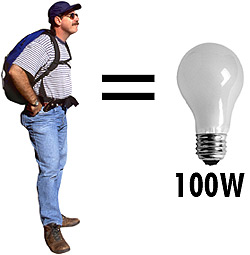QUESTION #420

# How much heat per hour do humans dissipate?This means that the average person expends ~8.37 x 106 joules of energy per day, since most of us are in some sort of equilibrium with our surroundings. Assuming most of this energy leaves us in the form of heat, I calculate that on average we radiate ~350,000 J of energy per hour. Since Watt is just Joules per second, this is roughly equal to energy given off by a 100 Watt light bulb!